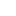v2.26IQuantity Interface Reference

Represents a quantity. More...

Inheritance diagram for IQuantity:## Public Member Functions

HRESULT HasValue ([out, retval] VARIANT_BOOL *pResult)
Indicates if the quantity has a value. More...

HRESULT AsCount ([out, retval] int *pValue)
Returns the quantity value as a number. More...

HRESULT AsLength ([in] enum LengthUnit unit, [out, retval] double *pValue)
Returns the quantity value as a length measure. More...

HRESULT AsArea ([in] enum AreaUnit unit, [out, retval] double *pValue)
Returns the quantity value as an area measure. More...

HRESULT AsVolume ([in] enum VolumeUnit unit, [out, retval] double *pValue)
Returns the quantity value as a volume measure. More...

HRESULT AsMass ([in] enum MassUnit unit, [out, retval] double *pValue)
Returns the quantity value as a mass measure. More...

## Properties

QuantityType Type` [get]`
The type of the quantity.

## Detailed Description

Represents a quantity.

Quantity contains the value of one of the following types: number, length, area, volume or mass. This interface can be obtained through IQuantityContainer.

## ◆ AsArea()

 HRESULT IQuantity::AsArea ( [in] enum AreaUnit unit, [out, retval] double * pValue )

Returns the quantity value as an area measure.

Parameters
 [in] unit The area measurement unit. [out] pValue The quantity's value in specified units.

## ◆ AsCount()

 HRESULT IQuantity::AsCount ( [out, retval] int * pValue )

Returns the quantity value as a number.

Parameters
 [out] pValue The quantity's value.

## ◆ AsLength()

 HRESULT IQuantity::AsLength ( [in] enum LengthUnit unit, [out, retval] double * pValue )

Returns the quantity value as a length measure.

Parameters
 [in] unit The length measurement unit. [out] pValue The quantity's value in specified units.

## ◆ AsMass()

 HRESULT IQuantity::AsMass ( [in] enum MassUnit unit, [out, retval] double * pValue )

Returns the quantity value as a mass measure.

Parameters
 [in] unit The mass measurement unit. [out] pValue The quantity's value in specified units.

## ◆ AsVolume()

 HRESULT IQuantity::AsVolume ( [in] enum VolumeUnit unit, [out, retval] double * pValue )

Returns the quantity value as a volume measure.

Parameters
 [in] unit The volume measurement unit. [out] pValue The quantity's value in specified units.

## ◆ HasValue()

 HRESULT IQuantity::HasValue ( [out, retval] VARIANT_BOOL * pResult )

Indicates if the quantity has a value.

Returns
TRUE if the quantity has a value; otherwise returns FALSE.